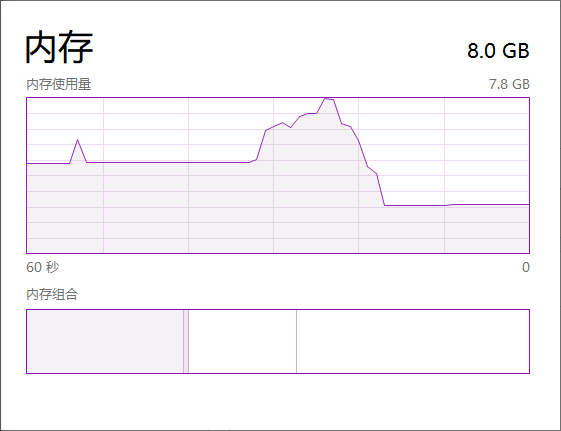# Tensorflow Dataset iterator consumes large amounts of memory

I am learning machine learning recently and try to implement a simple neural network using Tensorflow.

I use MNIST as my dataset, and I want to use Tensorflow's Dataset API to load and batch my data.

Here is my code:

```
train_data = tf.data.Dataset.from_tensor_slices((X_train, y_train))
train_data = train_data.shuffle(500)
train_data = train_data.batch(50)
train_data = train_data.repeat()
td_iter = train_data.make_one_shot_iterator()
features, labels = td_iter.get_next()

with tf.Session() as sess:
sess.run(init)
for epoch in range(n_epochs):
for iteration in range(n_batches):
X_batch, y_batch = sess.run([features, labels])
sess.run(training_op, feed_dict={X:X_batch, y:y_batch})
acc_train = accuracy.eval(feed_dict={X:X_batch, y:y_batch})
acc_test = accuracy.eval(feed_dict={X:X_test, y:y_test})
print(epoch, "Train accuracy:", acc_train, "Test accuracy:", acc_test)

```

I am able to train a model with high accuracy, but it costs all of my memory (8GB) when I train it.

More specific, it consumes large amounts of memory before finishing the first epoch (and it takes a rather long time to print the first output line), but the memory consumption is decreasing if it starts printing something.

I try to simplify the code to find out the problem:

```
with tf.Session() as sess:
sess.run(init)
sess.run([features, labels])

```

And the code above still eats up all my memory.I think it must be some mistake in my code, can you help me?

Thanks!

• Can you print the shapes of your X_train and y_train? Maybe the shuffle buffer occupies a lot of memory. Also, you do not actually need to feed_dict the data you get from tf data. You should be able to feed the features and labels directly since the graph would have created a node for these already. Here is an example from the repo that highlights this – kvish Feb 24 at 13:15
• @kvish The shape of X_train is (55000,784) and y_train is (55000, ), I am searching for a common practice of using Dataset API but cannot find a satisfied answer, thank you. – TomLeung Feb 24 at 13:29
• that is small amount of memory. One thing I had missed before is that if you are talking about GPU memory, then Tensorflow by default allocates all the memory but uses only as and when its required. If this is CPU RAM, I would also take a look at how you have defined your neural network. The graph that you are defining could be big and occupy lot of memory. Here is an example where that has happened – kvish Feb 24 at 14:02
• @kvish This is the RAM on my laptop's mainboard, and my NN is just a feed forward NN with 2 hidden layer, 300 units in the first layer and 200 in the second. The output layer has 10 units. – TomLeung Feb 24 at 14:11
• @kvish I remove `feed_dict` from my code, directly using X and y like your example (no placeholders). It obviously shorten the preparation time (before I saw the first epoch output) and reduce some memory usage. Do you think 0.8 ~ 1 GB is a normal consumption of MNIST dataset? – TomLeung Feb 24 at 14:28